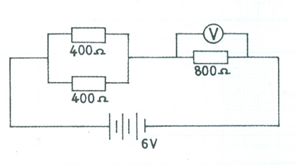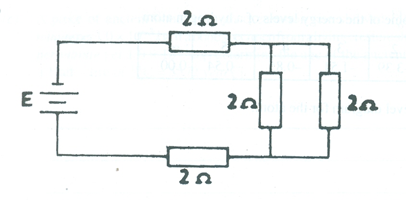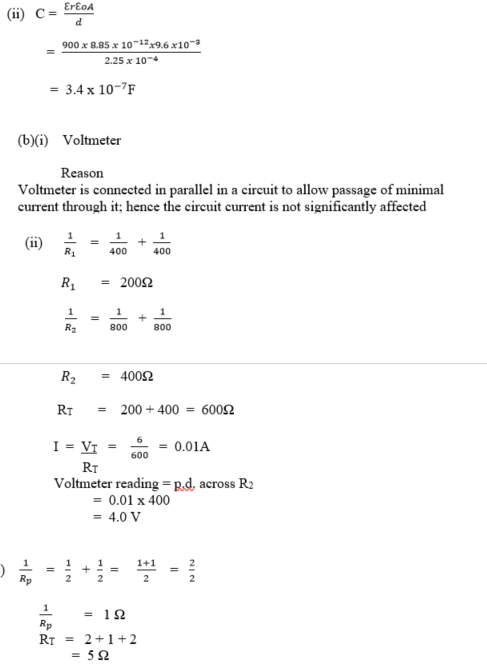Question 11

1. (i) What is dielectric?

(ii) A parallel plate capacitor consists of two plates each of area 9.6 x 10-3 m2, separated by dielectric of thickness 2.25 x 10-4 m and dielectric constant 900. Calculate the capacitance of the capacitor. [ℇo = permittivity of free space = 8.85 x 10-12 Fm-1].

1. (i) Which of the following devices has a higher resistance: an ammeter or a voltmeter? Give a reason for your answer.

(ii)The resistance of the voltmeter in the circuit diagram illustrated above is 800 Ω. Calculate the voltmeter reading.

(C)A battery of negligible internal resistance is connected to a set of resistors as illustrated in the circuit diagram above, Determine the equivalent resistance of the circuit.

Observation

Part (a)(i): Candidates performance was very fair. Most candidates were unable to correctly define the term dielectric.
(ii)  Majority of the candidates stated the formula correctly but could not make correct substitution. Performance was fair
Part (b)(i): Candidates performance was low. Candidates were unable to give a substantial reason while voltmeter has a higher resistance than ammeter.
(ii): Most candidates failed to take the given coil resistance of the voltmeter into consideration. Performance was low.
Part (c):     Performance was fair. Many candidates were able to calculate the weight of the oil drop using the formula W = qE. However, some got confused and used W = B/q.

Part (d):     Performance was fair. Many candidates were able to solve the problem correctly.